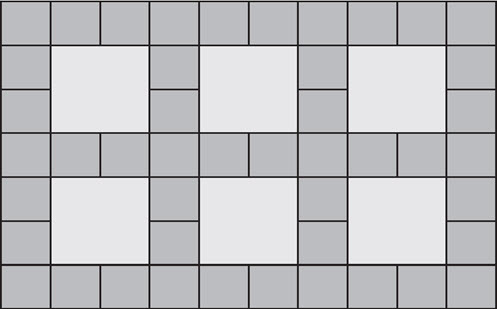Go to website

# reSolve: Multiplication: The Tiler

This task explores arrays through the context of a tiling a courtyard. Students are given the total cost of tiling a courtyard and use this to calculate the price for individual tiles. They then explore the cost of different tiling designs to determine if one is cheaper than another. Each lesson is outlined in detail including curriculum links, vocabulary, materials needed, sample answers, discussion points and student resources. This sequence is part of the reSolve: Mathematics by Inquiry program.

Year level(s) Year 4
Audience Teacher
Purpose Teaching resource
Teaching strategies and pedagogical approaches Mathematics investigation
Keywords division, multiplication, arrays

## Curriculum alignment

Curriculum connections Numeracy
Strand and focus Number, Build understanding, Apply understanding
Topics Multiplication and division
AC: Mathematics (V9.0) content descriptions
AC9M4N08
Use mathematical modelling to solve practical problems involving additive and multiplicative situations including financial contexts; formulate the problems using number sentences and choose efficient calculation strategies, using digital tools where appropriate; interpret and communicate solutions in terms of the situation

AC9M4A02
Recall and demonstrate proficiency with multiplication facts up to 10 x 10 and related division facts; extend and apply facts to develop efficient mental strategies for computation with larger numbers without a calculator

AC9M4N06
Develop efficient strategies and use appropriate digital tools for solving problems involving addition and subtraction, and multiplication and division where there is no remainder

Multiplicative strategies (P6, P7)
Number and place value (P7)
Understanding money (P7)